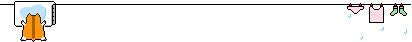### $\rm{0x01}$ 关于莫队### $\rm{0x02}$ 一些操作

    for(register int i = 1; i <= m; ++i)
{
while(l < q[i].l) dec(l++);
while(l > q[i].l) add(--l);
while(r < q[i].r) add(++r);
while(r > q[i].r) dec(r--);
//do something...
}### $\rm{0x03}$ 关于此题![](01)

![](02)